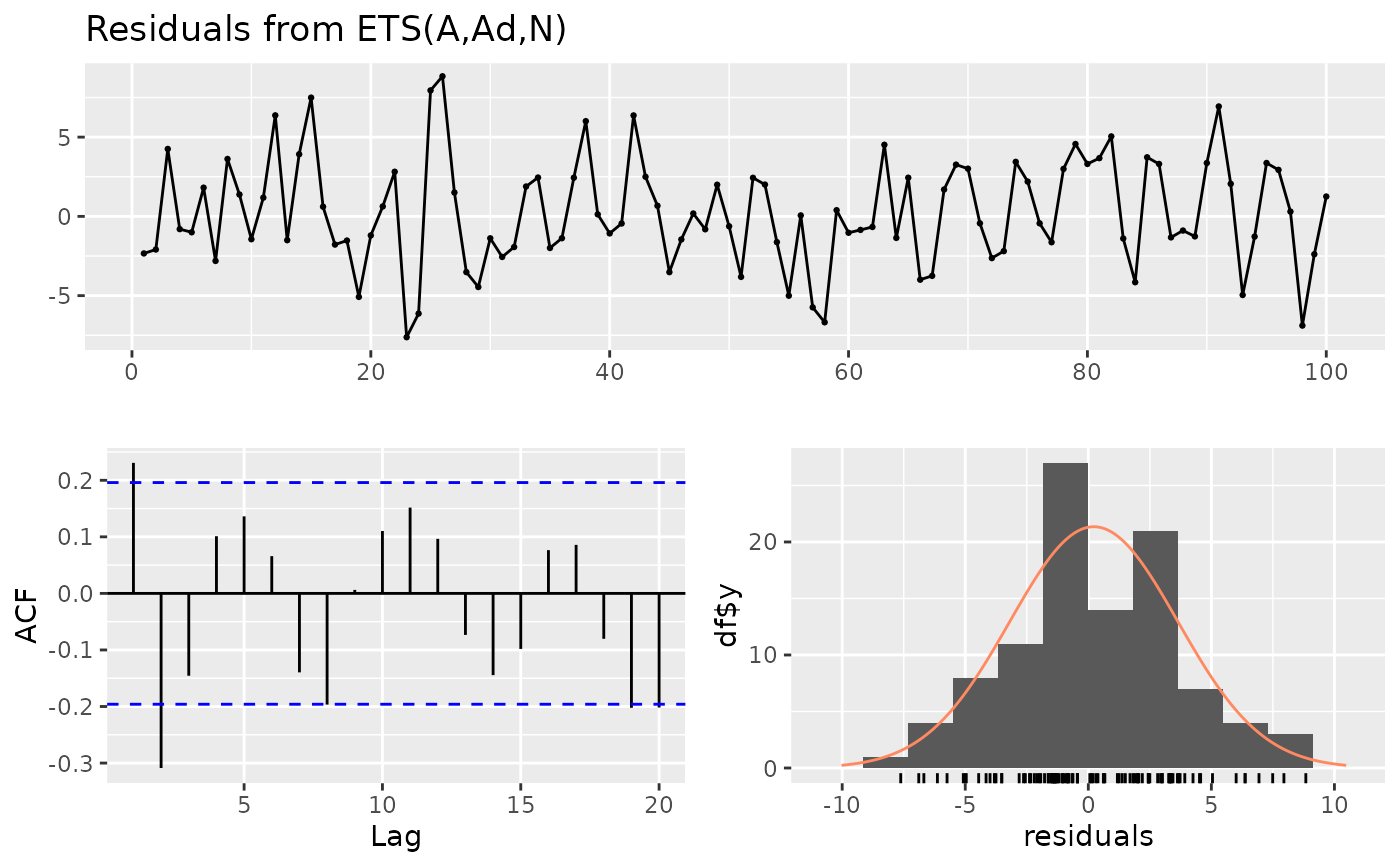If plot=TRUE, produces a time plot of the residuals, the corresponding ACF, and a histogram. If the degrees of freedom for the model can be determined and test is not FALSE, the output from either a Ljung-Box test or Breusch-Godfrey test is printed.

checkresiduals(object, lag, df = NULL, test, plot = TRUE, ...)

## Arguments

object Either a time series model, a forecast object, or a time series (assumed to be residuals). Number of lags to use in the Ljung-Box or Breusch-Godfrey test. If missing, it is set to min(10,n/5) for non-seasonal data, and min(2m, n/5) for seasonal data, where n is the length of the series, and m is the seasonal period of the data. It is further constrained to be at least df+3 where df is the degrees of freedom of the model. This ensures there are at least 3 degrees of freedom used in the chi-squared test. Number of degrees of freedom for fitted model, required for the Ljung-Box or Breusch-Godfrey test. Ignored if the degrees of freedom can be extracted from object. Test to use for serial correlation. By default, if object is of class lm, then test="BG". Otherwise, test="LB". Setting test=FALSE will prevent the test results being printed. Logical. If TRUE, will produce the plot. Other arguments are passed to ggtsdisplay.

## Value

None

ggtsdisplay, Box.test, bgtest

Rob J Hyndman

## Examples


fit <- ets(WWWusage)
checkresiduals(fit)#>
#> 	Ljung-Box test
#>